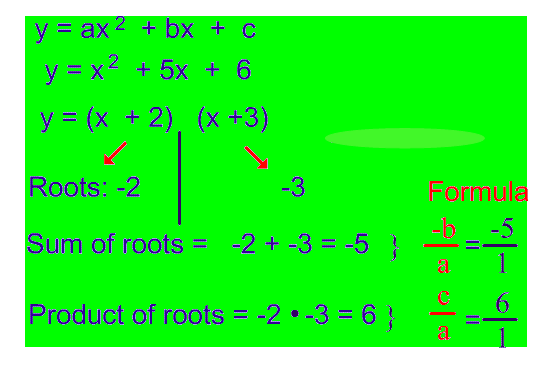### Quadratic Equation 2

Mathematics SSS 2 First Term

Theme: Numbers and Numeration

WEEK 7

Performance Objectives

Students should be able to;

1. Form a quadratic equation given the sum and the products of the root

2. Form quadratic equations from word problems

3. Solve word problems by solving suitable quadratic equations

Construction of Quadratic Equation from Sum and Product Roots

Content

From The Formulas

sum of roots: −XY−XY

product of roots: PqPq

From the work below, when you are trying to solve a quadratic equation in the form of ax2+bx+cax2+bx+c, the sum and product of the roots can be rewritten using the two formulas belowExample 1